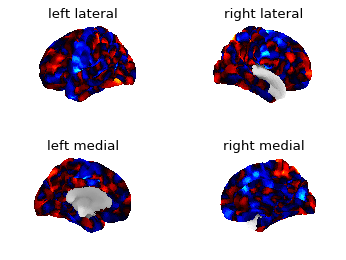# Preprocessed NKI fsaverage5 surface data

I was plotting the first time point of the functional data from the NKI preprocessed surface data with nilearn (0.5.0). The medial wall of the right hemisphere doesn’t seem right…

Here’s the code to replicate this proble:

``````from nilearn.datasets import fetch_surf_nki_enhanced, fetch_surf_fsaverage5
from nilearn.plotting import plot_surf_stat_map
import numpy as np
import matplotlib.pyplot as plt

nki = fetch_surf_nki_enhanced(1)
fs5 = fetch_surf_fsaverage5()

modes = ['lateral', 'medial']
hemis = ['left', 'right']

fig, axes = plt.subplots(nrows=len(modes), ncols=len(hemis),
figsize=(plt.figaspect(len(modes) / 2 * 0.75)), # not the best fix
subplot_kw={'projection': '3d'},
dpi=80)

for index_mode, mode in enumerate(modes):
for index_hemi, hemi in enumerate(hemis):
plot_surf_stat_map(fs5['pial_%s' %(hemi)],
stat_map=cur_statmap,
view=mode, hemi=hemi,
bg_map=fs5['sulc_%s' %(hemi)],
axes=axes[index_mode, index_hemi],
title=hemi+ ' ' + mode,
colorbar=False
)
``````

Out:I tried to plot the data of right hemisphere on the left hemisphere just to see what happens. It plots the medial view on the lateral part and vice versa.

I doubt there’s an issue in the code of nilearn. It could be the preprocessing? If so, how should we fix this?

hello, thank you for reporting this.

it seems that both files that nilearn downloads contain data for the left hemisphere; if you check you will see that ‘func_left’ and ‘func_right’ contain the same data,

point to the same file.

this is not a issue with nilearn but with the data that has been uploaded.

I opened an issue:

1 Like